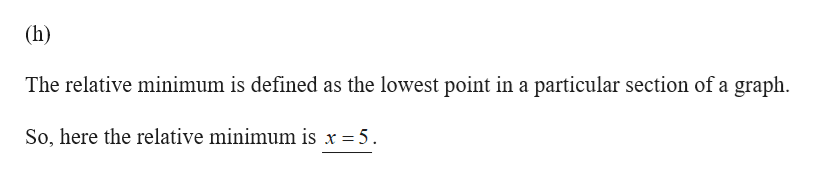# (a) the domain of f(b) the range of f(c) the x-intercepts(d) the y-intercept(e) intervals on which f is increasing(f) intervals on which f is decreasing(g) intervals on which f is constant(h) the number at which f has a relative minimum(i) the relative minimum of fU) f(2)(k) The values of x for which f(x) -3(l) Is feven, odd or neither?Use the graph to find the following.rtHetio00 mew9.2418-12-6te orX-6121071(a) What is the domain of f?(Type your answer in interval notation.)(b) What is the range of f?uwpesatntermme ldtezge00 10 ujs19 3r(Type your answer in interval notation.)odw(c) What are the zeros of the function?novs hationNEonThe left zero of the function is 3 and the right zero is(d) What is the y-intercept?The y-intercept of the function is(e) Over what interval is f increasing?055(Type your answer in interval notation.)eve narNen not.on(f) Over what interval is f decreasing?ww(Type your answer in interval notation.)(g) Over what interval is f constant?rexie(Type your answer in interval notation.)(h) What is the number at which f has a relative minimum?eot to the y-x14024 036sonston s aua(i) What is the relative minimum of f?() What is f(-2)?f(-2)=-3 is when x = 4.(k) What are the x-values where f(x)= -3? The leftmost x-value where f(x)-3?What is the rightmost x-value where f(x)(I) Is feven, odd, or neither?CO

Question
48 views

Will someone help me with this problem? Sub parts g,h,i

check_circle

Step 1
Step 2help_outlineImage Transcriptionclose(h) The relative minimum is defined as the lowest point in a particular section of a graph So, here the relative minimum is x = 5 fullscreen

### Want to see the full answer?

See Solution

#### Want to see this answer and more?

Solutions are written by subject experts who are available 24/7. Questions are typically answered within 1 hour.*

See Solution
*Response times may vary by subject and question.
Tagged in

### Other